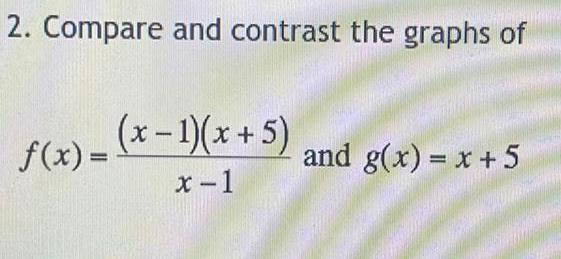Question:

# Compare and contrast the graphs of f(x) = (x - 1)(x + 5) /

Last updated: 7/4/2022Compare and contrast the graphs of f(x) = (x - 1)(x + 5) / (x-1) and g(x) = x + 5